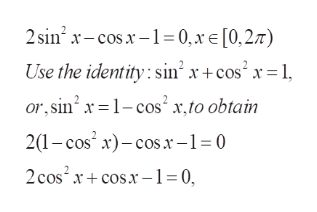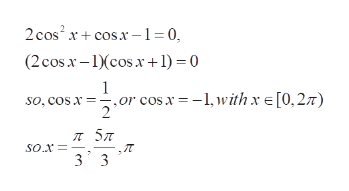Solvig hw 17

Question

Solvig hw 17

Step 1

To find all the values of x satisfying the given equation , with x in the interval [0,2pi)

Step 2

Using the standard identity, reduce the equation to a quadratic equation in cosxhelp_outlineImage Transcriptionclose2sin2 x-cosx1= 0, x E [0,27) Use the identity: sin x+ cos x=1 or, sin 1-cos'x,to obtain 21-cos x)cosx-1= 0 2cos2 xcos1 0, fullscreen
Step 3

Factorize the quadratic equation and solve for cos x,  and henc...help_outlineImage Transcriptionclose2cos2 xco-1=0, + (2cosx-cosx+ 1) = 0 cos.x-1,withx [0,27) so, cos x 57 so.x 3 3 fullscreen

Want to see the full answer?

See Solution

Want to see this answer and more?

Our solutions are written by experts, many with advanced degrees, and available 24/7

See Solution
Tagged in

Other